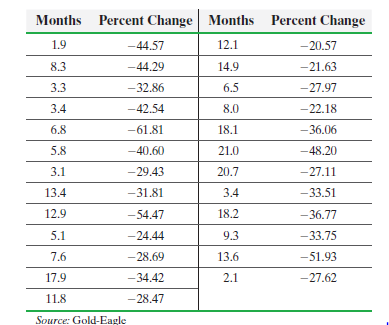×
Get Full Access to Statistics: Informed Decisions Using Data - 5 Edition - Chapter 14.1 - Problem 20
Get Full Access to Statistics: Informed Decisions Using Data - 5 Edition - Chapter 14.1 - Problem 20

×

# ?Bear Markets (Refer to Problem 32, Section 4.1) A bear market is a market condition in which the price of the security falls. A bear market in the stoISBN: 9780134133539 240

## Solution for problem 20 Chapter 14.1

Statistics: Informed Decisions Using Data | 5th Edition

• Textbook Solutions
• 2901 Step-by-step solutions solved by professors and subject experts
• Get 24/7 help from StudySoup virtual teaching assistantsStatistics: Informed Decisions Using Data | 5th Edition

4 5 1 389 Reviews
23
5
Problem 20

Bear Markets (Refer to Problem 32, Section 4.1) A bear market is a market condition in which the price of the security falls. A bear market in the stock market is defined as a condition in which market declines by 20% or more over the course of at least two months. The following data represent the number of months and percentage change in the S&P500 (a group of 500 stocks).(a) Treating months as the explanatory variable, x, determine the estimates for0 and1.

(b) Assuming the residuals are normally distributed, test whether a linear relation exists between the number of months of a bear market and percent change at the= 0.05 level of significance.

(c) Assuming the residuals are normally distributed, construct a 95% confidence interval for the slope of the true leastsquares regression line.

(d) Based on your results to parts (b) and (c), would you recommend using the least-squares regression line to predict the percent change in the S&P500 during a bear market? Why? What would be a good estimate of the percent change in the S&P500 during a bear market?

Step-by-Step Solution:

Step 1 of 5) Bear Markets (Refer to Problem 32, Section 4.1) A bear market is a market condition in which the price of the security falls. A bear market in the stock market is defined as a condition in which market declines by 20% or more over the course of at least two months. The following data represent the number of months and percentage change in the S&P500 (a group of 500 stocks). (a) Treating months as the explanatory variable, x, determine the estimates for 0 and 1. (b) Assuming the residuals are normally distributed, test whether a linear relation exists between the number of months of a bear market and percent change at the = 0.05 level of significance. (c) Assuming the residuals are normally distributed, construct a 95% confidence interval for the slope of the true leastsquares regression line. (d) Based on your results to parts (b) and (c), would you recommend using the least-squares regression line to predict the percent change in the S&P500 during a bear market Why What would be a good estimate of the percent change in the S&P500 during a bear market

Step 2 of 2

## Discover and learn what students are asking

Statistics: Informed Decisions Using Data : Estimating a Population Mean
?The procedure for constructing a t-interval is robust. Explain what this means.

Statistics: Informed Decisions Using Data : Inference on the Least-Squares Regression Model and Multiple Regression
?The following data represent the height (inches) of boys between the ages of 2 and 10 years. (a) Treating age as the explanatory variable, de

Unlock Textbook Solution